# Circles

For the circle c1(S1; r1=146 cm) and c2(S2; r2 = 144 cm) is distance of centers |S1S2| = 295 cm. Determine the distance between the circles.

Result

d =  5 cm

#### Solution:

$d = 295-146-144 = 5 \ \text{cm}$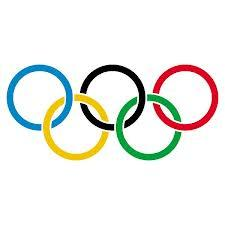Our examples were largely sent or created by pupils and students themselves. Therefore, we would be pleased if you could send us any errors you found, spelling mistakes, or rephasing the example. Thank you!

Leave us a comment of this math problem and its solution (i.e. if it is still somewhat unclear...):Be the first to comment!## Next similar math problems:

1. Lake or pondThe landlord has a square lake. Trees grow around this lake. The lake wants to enlarge the pond twice and does not want to cut down or flood any tree. How will he do that?
2. CagesHonza had three cages (black, silver, gold) and three animals (guinea pig, rat and puppy). There was one animal in each cage. The golden cage stood to the left of the black cage. The silver cage stood on the right of the guinea pig cage. The rat was in theAdded together and write as decimal number: LXVII + MLXIV
4. Plane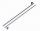On how many parts divide plane 6 parallels?
5. The resultHow many times I decrease the number 1632 to get the result 24?
6. If-then equationIf 5x - 17 = -x + 7, then x =
7. Ten pupils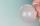10 pupils went to the store. 6 pupils bought lollipops and 9 pupils bought chewing gum. How many pupils have bought both lollipops and chewing gums (if everyone bought something)?
8. Eq1Solve equation: 4(a-3)=3(2a-5)
9. Line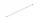How many parts of line divide 5 (different) points that lie on it?
10. Expressions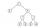Find out value of expressions if a = -1, b =2: x=b - 2a - ab y=a3 - b2 - 2ab z=a2 b3 - a3 b2 w=a + b + a3 - b2
11. One frame5 picture frames cost € 12 more than three frames. How much cost one frame?
12. Six te 2If 3t-7=5t, then 6t=
13. Valid numberRound the 453874528 on 2 significant numbers.
14. Flood waterFlood waters in some US village meant that the homes had to evacuate 364 people. 50 of them stayed at elementary schools, 59 them slept with their friends and others went to relatives. How many people have gone to relatives?
15. Math classificationIn 3A class are 27 students. One-third got a B in math and the rest got A. How many students received a B in math?
16. CakesOn the bowl were a few cakes. Jane ate one-third of them, Dana ate a quarter of those cakes that remained. a) What part (of the original number of cakes) Dana ate? b) At least how many cakes could be (initially) on thebowl?
17. Italian writerThere were 17 children in the barracks with an Italian writer. 6 children ordered a book in an Italian original and 12 children in the translation. How many children ordered a book in both languages if the three children did not order it?